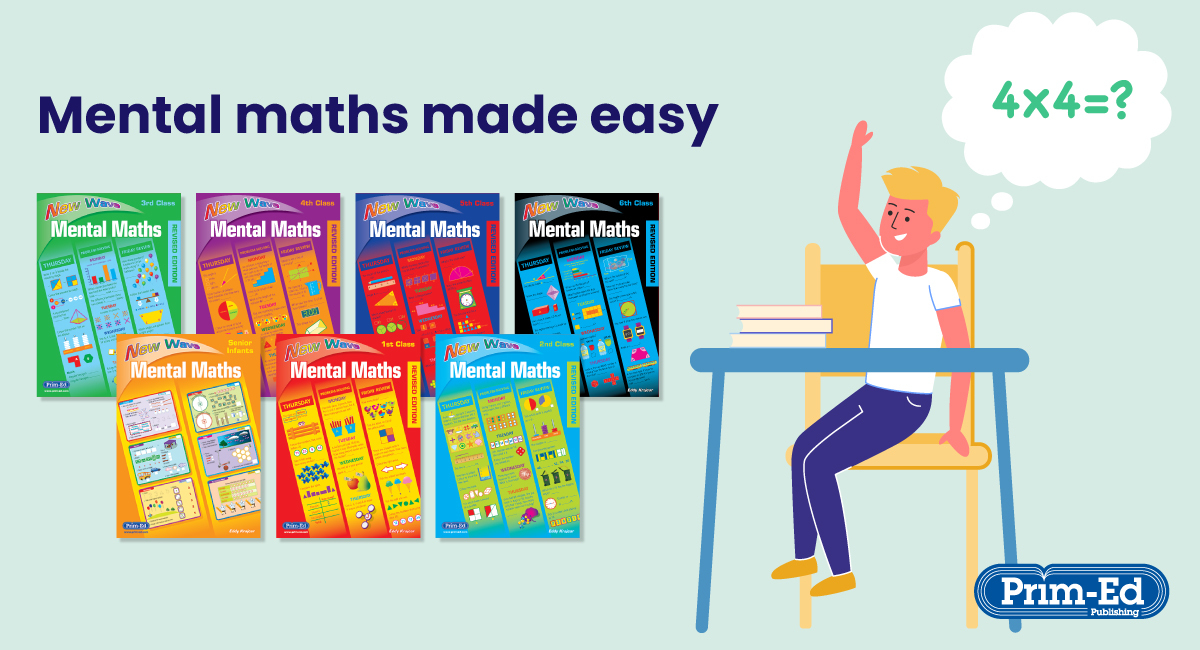• Wednesday 04 August 2021

Mental maths can be daunting for children, and hey, sometimes it’s tricky for us adults too! Luckily, there are some methods that can make mental maths easier to learn and apply. Read on for a few mental maths tips and tricks to help your children master their mental arithmetic in no time. You’ll wish you’d known them in school!

##### 1. Doubling large numbers
• Multiply each number by 2 and add them together.
As an example: 243 x 2
200 x 2 = 400
40 x 2 = 80
3 x 2 = 6
400 + 80 + 6 = 486
##### 2. Multiplying 2-digit numbers by 11
• Add the two digits together and place the result in the middle! How easy is that?
As an example: 34 x 11
3 + 4 = 7
##### 3. Multiplying by 9
• Multiply the number by 10, then subtract the original number from the total.
As an example: 47 x 9
47 x 10 = 470
470 – 47 = 423
##### 4. Multiplying decimals by 10, 100, 1000
• To multiply a number by 10, move the decimal point once to the right.
E.g. 45.725 x 10 = 457.25
To multiply a number by 100, move the decimal point twice to the right.
E.g. 45.725 x 100 = 4572.5
To multiply a number by 1000, move the decimal point three times to the right.
E.g. 45.725 x 1000 = 45 725
##### 5. Dividing decimals by 10, 100, 1000
• To divide a number by 10, move the decimal point once to the left.
E.g. 6292.3 ÷ 10 = 629.23
To divide a number by 100, move the decimal point twice to the left.
E.g. 6292.3 ÷ 100 = 62.923
To divide a number by 1000, move the decimal point three times to the left.
E.g. 6292.3 ÷ 1000 = 6.2923

##### Put these tips and tricks into practice!

New Wave Mental Maths workbooks are perfect for children to reinforce, practice and consolidate their learning. This series provides daily maths practice to increase pupils’ fluency and automaticity across all maths strands. There’s 200 days’ of practice with a Friday Review every week. For the more confident mathematicians, they can have a go at testing their mental maths recall speed.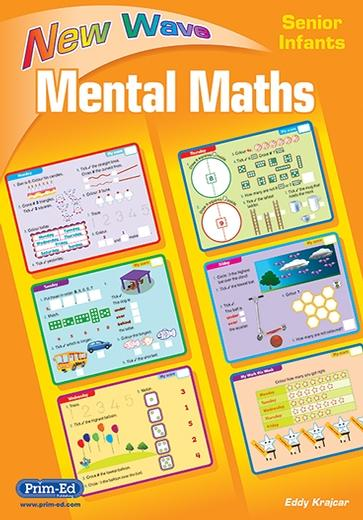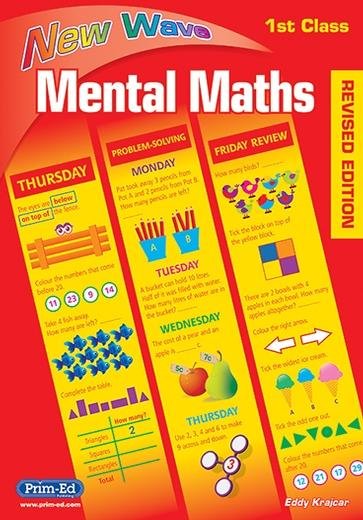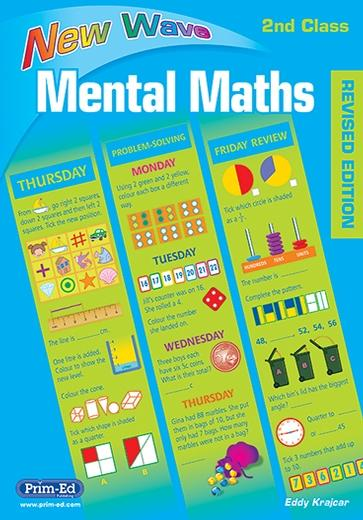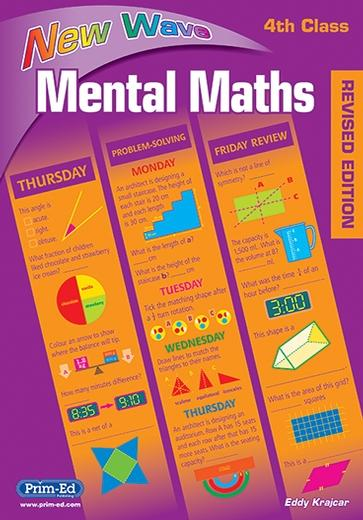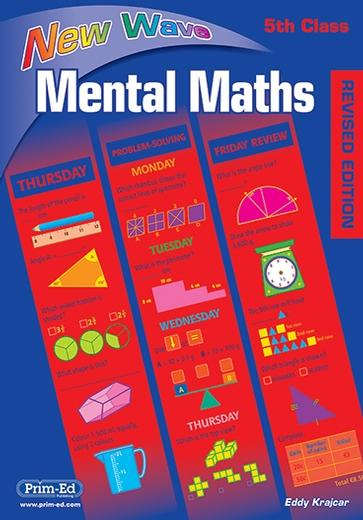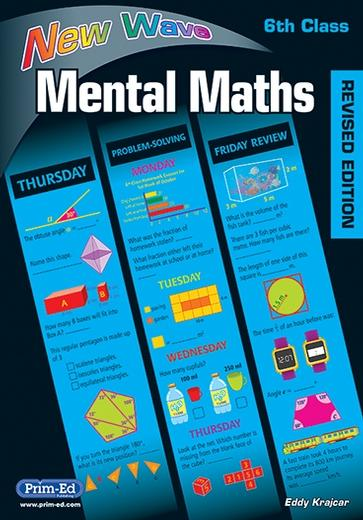Along with the workbooks, there's the New Wave Mental Maths online portal for teachers, complimentary with a class set of the workbooks.

With the portal you can:

1. display the daily workbook on the big screen, with the option to turn the answers on and off.
2. quickly recap concepts tied to tricky questions and retackle questions together.
3. track progression through the term-based assessment.

##### What have teachers and parents said about New Wave Mental Maths?

Kirsty, teacher

One of my absolute favourite resources to use in the classroom!

Jac, teacher

These are fantastic for differentiation and revision in the classroom. I use them all the time.

Mrs. Jones, teacher

Love these books! We use this as early morning maths work everyday! Kids love them.

Paarul P, parent

This is a favourite in our home and great for daily rigour. My child loves this workbook and I never hear any complaints. The format is nice and not overwhelming.